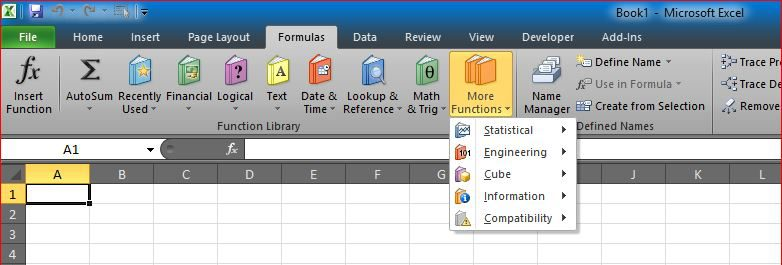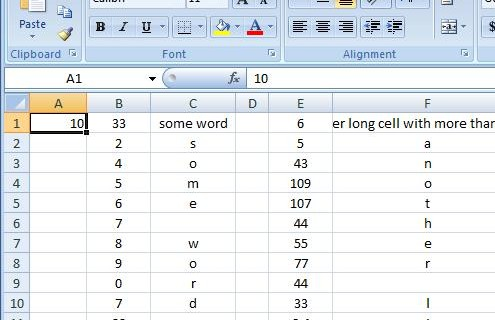# Fx to cut long column into two columnsConvert one long column into two
This set of formulas will get the items after row 45 (for example) from column A into the column B starting from B1 to have two 45-rows next to each other instead of one 90-rows column

This was the answer for this question
`=OFFSET(OneCol!\$B\$1,((INT((ROW()-1)/\$A\$1)+1)-1)*\$A\$1+ROW()-1,0)`
`=OFFSET(OneCol!\$B\$1,((INT((ROW()-1)/\$A\$1)+1))*\$A\$1+ROW()-1,0)`